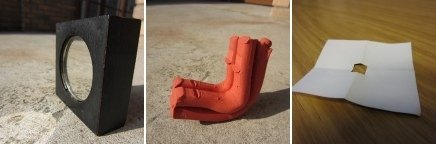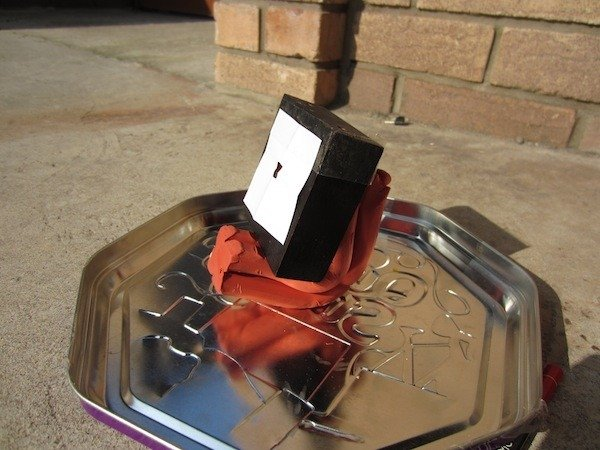Also available in italiano

# Measure the Solar Diameter

Created: 2013-10-01
Author(s): Edward Gomez, LCOGTGoals
• Students will have an appreciation of the relative and actual size of the Sun.
• Students will have an appreciation of how fast the Sun moves across the sky.Learning Objectives
• Students will be able to describe perspective which makes the Sun appear smaller in the sky than it is in reality.
• Students will be able to describe why the Sun appears to move across the sky when in fact its apparent motion is produced by Earth’s rotation.
• Students will be able to convert between different time units, angles and calculate an accurate diameter for the Sun.Background
• Understanding of the concept of angles and angular distance
• Understanding of the concept of velocity
• Appreciation that the sun's movement is apparent, and caused by the Earth's rotation, and
• Awareness of the dangers of looking directly at the Sun.Materials
• a small mirror (it needs to be covered by the thick paper, so it must be between 5 and 20 cm)
• a piece of thick paper or card to cover your mirror
• a tripod or a lump of Plasticine or playdough
• something to use as a screen (a piece of A4 or A3 white paper with a cardboard backing will do)
• a pencil or marker pen to write on the screen
• a stop watchFull Description

#### Setup:

1. Cut a small hole (something between a pinhole and 5mm diameter hole) in the centre of the piece of thick paper.
2. Tape this piece of paper to the front of your mirror, so that you can only see the mirror through the small hole you made.
3. Attach the mirror to the tripod, so that the paper faces upward.
4. If you don’t have a tripod, put the plasticine/playdough on the floor and embed the block on it so that the mirror is at about a 40 degree angle (facing the Sun).
5. Stand your screen up and make sure it cannot move during the experiment: if it does move, you will have to start all over again.#### Method:

1. Angle the mirror so the Sun is reflected onto the screen.
2. The projected image of the Sun must be circular, so angle your mirror and screen until it is.
3. Trace around the image of the Sun on the screen.
5. Wait until the Sun has moved to just outside the circle you drew.
6. Note the time on the stopwatch and reset.
7. Repeat steps 2–5 a few times (repeat 3–4 times for more accurate results).
8. Take the mean average of all your timings (in seconds).

#### Discussion and Results:

In this activity, you have been measuring how long it takes the Sun to move a distance equal to its own diameter across the sky. The Sun will take 24 hours to travel 360 degrees all the way around the sky and return to the same position it was in on the previous day. The speed it travels at is:

360 degrees/24hours:
= 360 deg/(24x60) minutes
= 0.25 deg per minute
= 0.00416 degrees per second or 1/240 degs per second

Calculate the size of the Sun as an angle: Average duration (in seconds) × 1/240 (degrees per second) = _ _ _ degrees

Congratulations! You have calculated the angular size of the Sun.

#### Calculate the physical size of the Sun

You can use your value for the angular size of the Sun to calculate the physical size of the Sun.

Your number for angular size converted into radians × distance from Earth to the Sun = size of the Sun

Angular size × (pi/180) × 92 955 887.6 miles = _ _ _ miles OR

Angular size × (pi/180) × 149 598 000 km = _ ______km

Congratulations! You have measured and calculated the diameter of the Sun in miles/kilometers!Evaluation
• Make sure each of the objectives is reached.
• Ask students to describe what is happening as Sun moves across sky by using a ball and globe (representing the Sun and Earth).
• Ask students to explain their measurements in terms of the ball and globe model.Curriculum
Country Level Subject Exam Board Section
UK GCSE Physics AQA Science A Not in current curriculum
UK GCSE Physics Edexcel P1.3.4
UK GCSE Physics OCR A P7.1: 1-4
UK GCSE Physics OCR B Not in current curriculum
UK GCSE Physics WJEC Not in current curriculum
UK GCSE Astrophysics Edexcel Unit 1.1.3: a, b
UK KS3 Physics - Space Physics: Our Sun as a StarAdditional Information
• Make sure you are getting an image of the Sun reflected from the mirror and not just a reflection of the mirror itself. The small hole in the thick paper is essential in this regard. If the hole is too big or the paper is too far from the mirror, you will not get a good projection. This will take some experimentation.
• Make sure the screen does not accidently get moved.
• Make sure the screen is face on to the mirror so the projected image is circular.
• The Sun does not take exactly 24 hours to return to the same place in the sky because in that time, the Earth has moved a 1/365th of its journey around the Sun. This phenomenon is masked by the measurement accuracy of this activity, but you might like to discuss it with the students.
• When performing the calculation we convert 360° into 2pi radians. Radians allow us to make calculations involving angles and distances. It can be explained to the students by saying that degrees are a unit. When you walk in a circle, although you have moved 360° you have also walked 2pi x radius of the circle. You can prove this by drawing a small circle on paper, wrapping a piece of string around the edge and then measuring the string. The measurement will be the same as 2pi x radius of the circle you drew.
• You can see a full calculation of the diameter worked through as an interactive pencast on the LCOGT website http://lcogt.net/education/activity/measure-diameter-sunConclusion

The activity concludes when all the steps have been completed and a size for the Sun has been calculated (and compared with a value listed in a text book). If there are multiple groups doing this activity, collect all the answers and see how much variation there is.

This activity can be used to start a discussion about many other astronomical concepts, such as size scales in the Solar System or unit conversions.